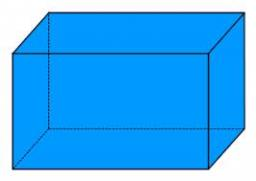# Cuboid

Cuboid with edge a=6 cm and body diagonal u=31 cm has volume V=900 cm3. Calculate the length of the other edges.

Correct result:

b =  30 cm
c =  5 cm

#### Solution:

$a=6 \ \text{cm} \ \\ V=900 \ \text{cm}^3 \ \\ u=31 \ \text{cm} \ \\ \ \\ V=abc \ \\ 900=6 \ bc \ \\ bc=150 \ \\ \ \\ u=\sqrt{ a^2+b^2+c^2 } \ \\ \ \\ 31^2=6^2 + b^2 + c^2 \ \\ \ \\ 925=b^2 + c^2 \ \\ 925=\dfrac{ 22500 }{ c^2 } + c^2 \ \\ c^4-925 \ c^2 + 22500=0 \ \\ x=c^2 \ \\ \ \\ x^2-925x + 22500=0 \ \\ \ \\ x^2 -925x +22500=0 \ \\ \ \\ a=1; b=-925; c=22500 \ \\ D=b^2 - 4ac=925^2 - 4\cdot 1 \cdot 22500=765625 \ \\ D>0 \ \\ \ \\ x_{1,2}=\dfrac{ -b \pm \sqrt{ D } }{ 2a }=\dfrac{ 925 \pm \sqrt{ 765625 } }{ 2 } \ \\ x_{1,2}=\dfrac{ 925 \pm 875 }{ 2 } \ \\ x_{1,2}=462.5 \pm 437.5 \ \\ x_{1}=900 \ \\ x_{2}=25 \ \\ \ \\ \text{ Factored form of the equation: } \ \\ (x -900) (x -25)=0 \ \\ \ \\ c_{1}=\sqrt{ x_{1} }=\sqrt{ 900 }=30 \ \\ c_{2}=\sqrt{ x_{2} }=\sqrt{ 25 }=5 \ \\ \ \\ b=c_{1}=30 \ \text{cm}$

Checkout calculation with our calculator of quadratic equations.Our examples were largely sent or created by pupils and students themselves. Therefore, we would be pleased if you could send us any errors you found, spelling mistakes, or rephasing the example. Thank you!

Please write to us with your comment on the math problem or ask something. Thank you for helping each other - students, teachers, parents, and problem authors.Tips to related online calculators
Looking for help with calculating roots of a quadratic equation?
Do you have a system of equations and looking for calculator system of linear equations?
Do you want to convert length units?
Tip: Our volume units converter will help you with the conversion of volume units.
Pythagorean theorem is the base for the right triangle calculator.

#### You need to know the following knowledge to solve this word math problem:

We encourage you to watch this tutorial video on this math problem:

## Next similar math problems:

• Solid cuboidA solid cuboid has a volume of 40 cm3. The cuboid has a total surface area of 100 cm squared. One edge of the cuboid has length 2 cm. Find the length of a diagonal of the cuboid. Give your answer correct to 3 sig. Fig.
• Block or cuboidThe wall diagonals of the block have sizes of √29cm, √34cm, √13cm. Calculate the surface and volume of the block.
• Faces diagonalsIf the diagonals of a cuboid are x, y, and z (wall diagonals or three faces) respectively than find the volume of a cuboid. Solve for x=1.3, y=1, z=1.2
• Three faces of a cuboidThe diagonal of three faces of a cuboid are 13,√281 and 20 units. Then the total surface area of the cuboid is.
• A bridgeA bridge over a river is in the shape of the arc of a circle with each base of the bridge at the river's edge. At the center of the river, the bridge is 10 feet above the water. At 27 feet from the edge of the river, the bridge is 9 feet above the water.
• An equilateralAn equilateral triangle is inscribed in a square of side 1 unit long so that it has one common vertex with the square. What is the area of the inscribed triangle?
• Sphere from tree pointsEquation of sphere with three point (a,0,0), (0, a,0), (0,0, a) and center lies on plane x+y+z=a
• Diagonal 20Diagonal pathway for the rectangular town plaza whose length is 20 m longer than the width. if the pathway is 20 m shorter than twice the width. How long should the pathway be?
• Diamond diagonalsCalculate the diamond's diagonal lengths if its content is 156 cm2 and the side length is 13 cm.
• Find the 13Find the equation of the circle inscribed in the rhombus ABCD where A[1, -2], B[8, -3] and C[9, 4].
• Three parallelsThe vertices of an equilateral triangle lie on 3 different parallel lines. The middle line is 5 m and 3 m distant from the end lines. Calculate the height of this triangle.
• Sides of right angled triangleOne leg is 1 m shorter than the hypotenuse, and the second leg is 2 m shorter than the hypotenuse. Find the lengths of all sides of the right-angled triangle.
• Two chordsCalculate the length of chord AB and perpendicular chord BC to circle if AB is 4 cm from the center of the circle and BC 8 cm from the center of the circle.
• Touch x-axisFind the equations of circles that pass through points A (-2; 4) and B (0; 2) and touch the x-axis.
• AP RT triangleThe length of the sides of a right triangle form an arithmetic progression, longer leg is 24 cm long. What are the perimeter and area?
• Right triangle eq2Find the lengths of the sides and the angles in the right triangle. Given area S = 210 and perimeter o = 70.
• Isosceles trapezoidCalculate the content of an isosceles trapezoid whose bases are at ratio 5:3, the arm is 6cm long and it is 4cm high.